bar

•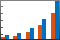Description

example

bar(y) creates a bar graph with one bar for each element in y. If y is an m-by-n matrix, then bar creates m groups of n bars.

example

bar(x,y) draws the bars at the locations specified by x.

example

bar(___,width) sets the relative bar width, which controls the separation of bars within a group. Specify width as a scalar value. Use this option with any of the input argument combinations in the previous syntaxes.

example

bar(___,style) specifies the style of the bar groups. For example, use 'stacked' to display each group as one multicolored bar.

example

bar(___,color) sets the color for all the bars. For example, use 'r' for red bars.

example

bar(___,Name,Value) specifies properties of the bar graph using one or more name-value pair arguments. Only bar graphs that use the default 'grouped' or 'stacked' style support setting bar properties. Specify the name-value pair arguments after all other input arguments. For a list of properties, see Bar Properties.

example

bar(ax,___) plots into the axes specified by ax instead of into the current axes (gca). The option ax can precede any of the input argument combinations in the previous syntaxes.

example

b = bar(___) returns one or more Bar objects. If y is a vector, then bar creates one Bar object. If y is a matrix, then bar returns a Bar object for each series. Use b to set properties of the bars after displaying the bar graph.

Examples

collapse all

y = [75 91 105 123.5 131 150 179 203 226 249 281.5];
bar(y)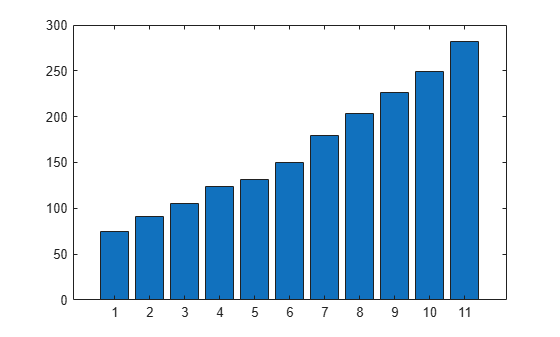Specify the bar locations along the x-axis.

x = 1900:10:2000;
y = [75 91 105 123.5 131 150 179 203 226 249 281.5];
bar(x,y)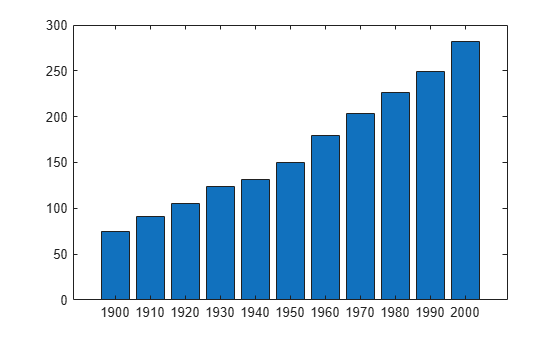Set the width of each bar to 40 percent of the total space available for each bar.

y = [75 91 105 123.5 131 150 179 203 226 249 281.5];
bar(y,0.4)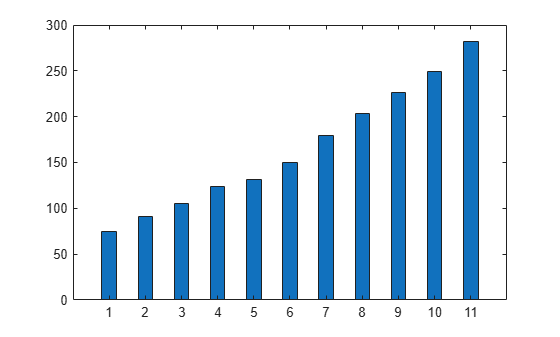Display four groups of three bars.

y = [2 2 3; 2 5 6; 2 8 9; 2 11 12];
bar(y)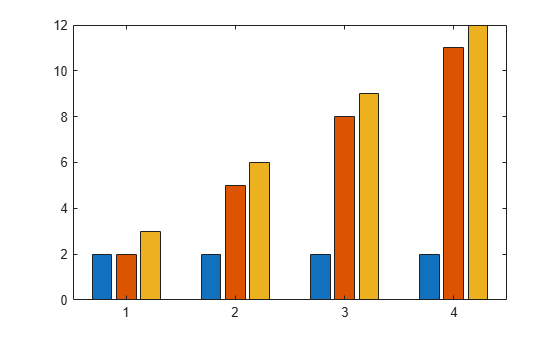Display one bar for each row of the matrix. The height of each bar is the sum of the elements in the row.

y = [2 2 3; 2 5 6; 2 8 9; 2 11 12];
bar(y,'stacked')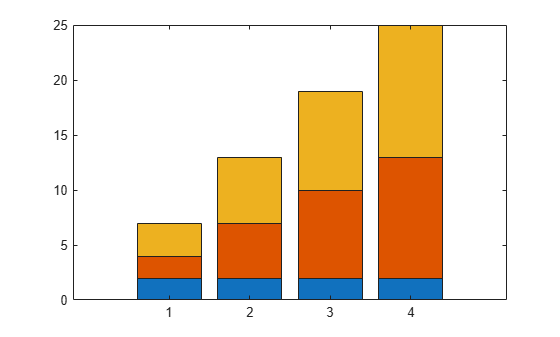Define x as a vector of three year values. Define y as a matrix that contains a combination of negative and positive values. Display the values in a bar graph.

x = [1980 1990 2000];
y = [15 20 -5; 10 -17 21; -10 5 15];
bar(x,y,'stacked')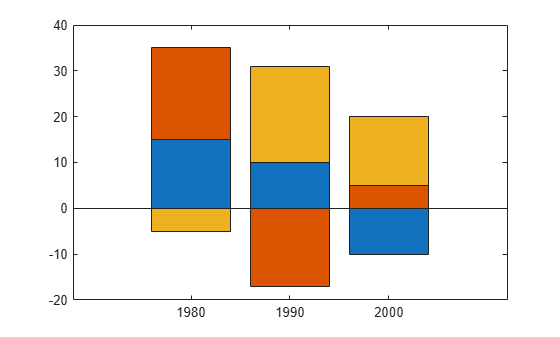One way to indicate categories for your bars is to specify X as a categorical array. The bar function uses a sorted list of the categories, so the bars might display in a different order than you expect. To preserve the order, call the reordercats function.

Define X as categorical array, and call the reordercats function to specify the order for the bars. Then define Y as a vector of bar heights and display the bar graph.

X = categorical({'Small','Medium','Large','Extra Large'});
X = reordercats(X,{'Small','Medium','Large','Extra Large'});
Y = [10 21 33 52];
bar(X,Y)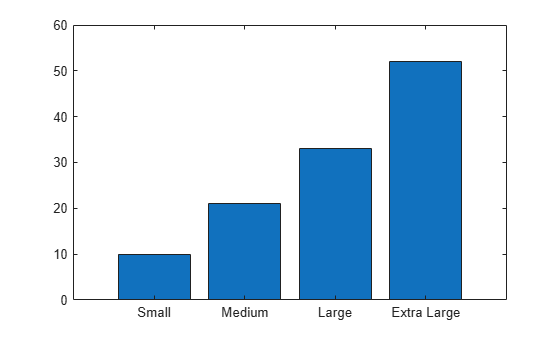Define vals as a matrix containing the values of two data sets. Display the values in a bar graph and specify an output argument. Since there are two data sets, bar returns a vector containing two Bar objects.

x = [1 2 3];
vals = [2 3 6; 11 23 26];
b = bar(x,vals);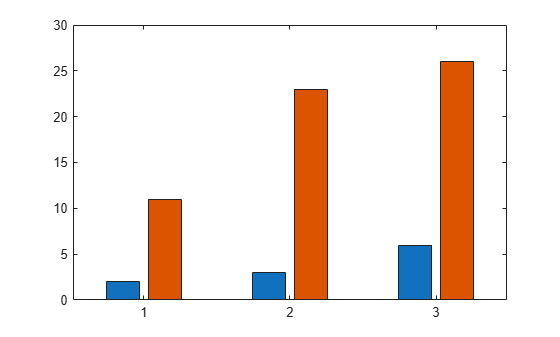Display the values at the tips of the first series of bars. Get the coordinates of the tips of the bars by getting the XEndPoints and YEndPoints properties of the first Bar object. Pass those coordinates to the text function, and specify the vertical and horizontal alignment so that the values are centered above the tips of the bars.

xtips1 = b(1).XEndPoints;
ytips1 = b(1).YEndPoints;
labels1 = string(b(1).YData);
text(xtips1,ytips1,labels1,'HorizontalAlignment','center',...
'VerticalAlignment','bottom')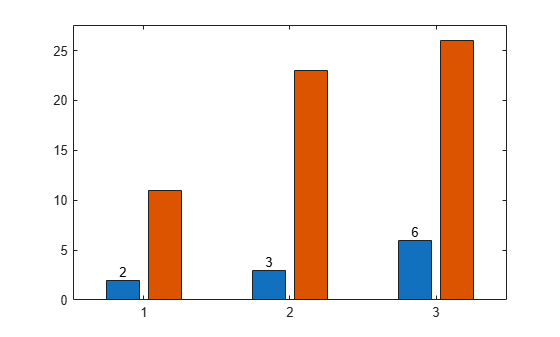Next, display the values above the tips of the second series of bars.

xtips2 = b(2).XEndPoints;
ytips2 = b(2).YEndPoints;
labels2 = string(b(2).YData);
text(xtips2,ytips2,labels2,'HorizontalAlignment','center',...
'VerticalAlignment','bottom')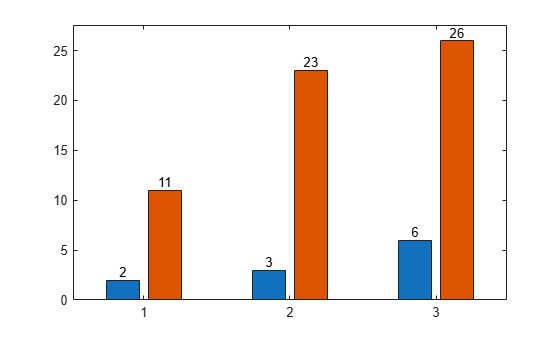Starting in R2019b, you can display a tiling of bar graphs using the tiledlayout and nexttile functions. Call the tiledlayout function to create a 2-by-1 tiled chart layout. Call the nexttile function to create the axes objects ax1 and ax2. Display a bar graph in the top axes. In the bottom axes, display a stacked bar graph of the same data.

y = [1 2 3; 4 5 6];
tiledlayout(2,1)

% Top bar graph
ax1 = nexttile;
bar(ax1,y)

% Bottom bar graph
ax2 = nexttile;
bar(ax2,y,'stacked')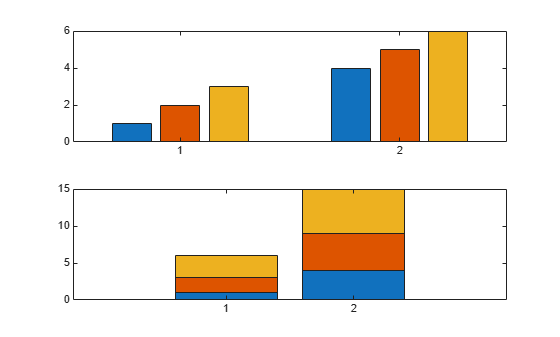Create a bar graph using red bars.

y = [75 91 105 123.5 131 150 179 203 226 249 281.5];
bar(y,'r')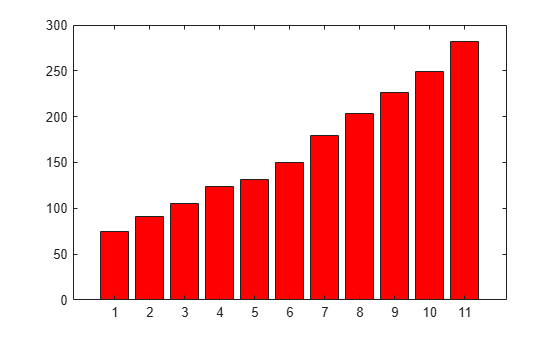Set the bar interior color and outline color using RGB triplets. Set the width of the bar outline.

y = [75 91 105 123.5 131 150 179 203 226 249 281.5];
bar(y,'FaceColor',[0 .5 .5],'EdgeColor',[0 .9 .9],'LineWidth',1.5)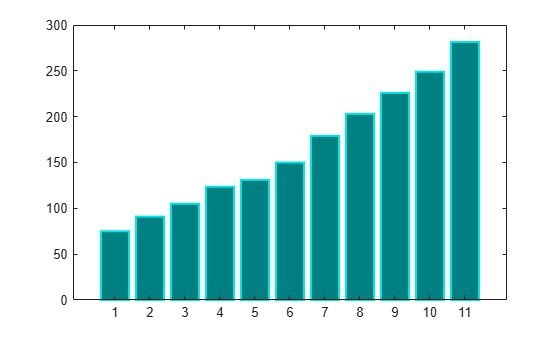Control individual bar colors using the CData property of the Bar object.

Create a bar chart and assign the Bar object to a variable. Set the FaceColor property of the Bar object to 'flat' so that the chart uses the colors defined in the CData property. By default, the CData property is prepopulated with a matrix of the default RGB color values. To change a particular color, change the corresponding row in the matrix. For example, change the color of the second bar.

b = bar(rand(10,1));
b.FaceColor = 'flat';
b.CData(2,:) = [.5 0 .5];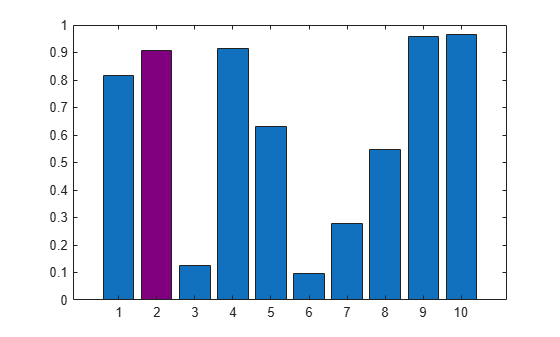Create a bar chart that uses colormap colors by setting the FaceColor property to 'flat'. Then set the CData property for each Bar object to an integer.

y = [1 3 5; 3 2 7; 3 4 2];
b = bar(y,'FaceColor','flat');
for k = 1:size(y,2)
b(k).CData = k;
end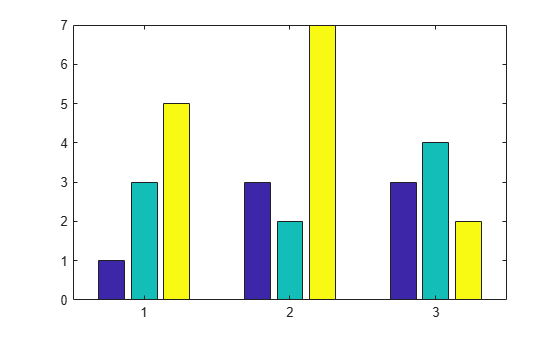Create matrix y, where each column is a series of data. Call the bar function to display the data in a bar graph, and specify an output argument. The output is a vector of three Bar objects, where each object corresponds to a different series. This is true whether the bars are grouped or stacked.

y = [10 15 20; 30 35 40; 50 55 62];
b = bar(y);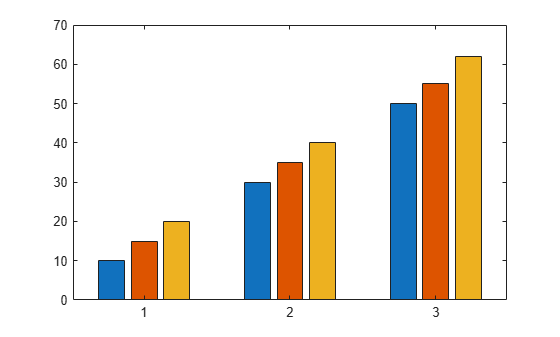Make the third series of bars green.

b(3).FaceColor = [.2 .6 .5];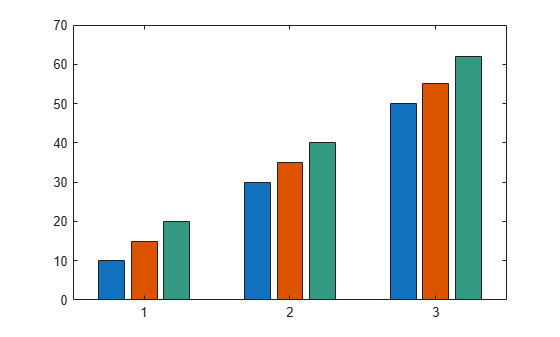Input Arguments

collapse all

x-coordinates, specified as a scalar, vector, or matrix. The values of x do not need to be in order, but the size of x depends on the size of y and how you want to display your data. This table describes the most common situations.

PresentationHow to Specify X and YExample
Display one series of bars.

Specify x and y as vectors that are the same length. The values in x must be unique, but the values in y do not need to be unique.

x = [1980 1990 2000];
y = [10 20 30];
bar(x,y)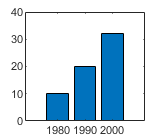Display multiple series of bars in groups.

Specify either of these combinations:

• Specify x and y as matrices of equal size. Each column of y corresponds to a series of bars. By default, each series is a different color. To ensure consistent placement of the groups, specify the columns of x as identical vectors. The values within a column must be unique, even though the columns are repeated.

• Specify x as a vector of unique values, and specify y as a matrix. The length of x must equal the length of at least one dimension of y. The other dimension of y contains values for the different series of bars.

x = [1980 1980 1980
1990 1990 1990];
y = [2 6 9
11 22 32];
bar(x,y)
Or
x = [1980 1990];
y = [2 6 9
11 22 32];
bar(x,y)Display one group of bars centered at one x value.

Specify x as a scalar and y as a vector.

x = 1990;
y = [10 20 30];
bar(x,y)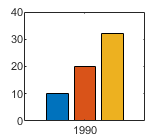Data Types: single | double | int8 | int16 | int32 | int64 | uint8 | uint16 | uint32 | uint64 | categorical | datetime | duration

y-coordinates, specified as a scalar, vector, or matrix. The size of y depends on the size of x and how you want to display your data. This table describes the most common situations.

PresentationHow to Specify X and YExample
Display one series of bars.

Specify x and y as vectors that are the same length. The values in x must be unique, but the values in y do not need to be unique.

x = [1980 1990 2000];
y = [10 20 30];
bar(x,y)Display multiple series of bars in groups.

Specify either of these combinations:

• Specify x and y as matrices of equal size. Each column of y corresponds to a series of bars. By default, each series is a different color. To ensure consistent placement of the groups, specify the columns of x as identical vectors. The values within a column must be unique, even though the columns are repeated.

• Specify x as a vector of unique values, and specify y as a matrix. The length of x must equal the length of at least one dimension of y. The other dimension of y contains values for the different series of bars.

x = [1980 1980 1980
1990 1990 1990];
y = [2 6 9
11 22 32];
bar(x,y)
Or
x = [1980 1990];
y = [2 6 9
11 22 32];
bar(x,y)Display one group of bars centered at one x value.

Specify x as a scalar and y as a vector.

x = 1990;
y = [10 20 30];
bar(x,y)Data Types: single | double | int8 | int16 | int32 | int64 | uint8 | uint16 | uint32 | uint64 | duration

Bar width, specified as a fraction of the total space available for each bar. The default of 0.8 means the bar width is 80% of the space from the previous bar to the next bar, with 10% of that space on each side.

If the width is 1, then the bars within a group touch one another.

Example: bar([1 2 3],0.5) creates bars that use 50% of the available space.

Data Types: single | double | int8 | int16 | int32 | int64 | uint8 | uint16 | uint32 | uint64

Group style, specified by one of these values.

StyleResultExample

'grouped'

Display each group as adjacent bars that are centered around their corresponding x value.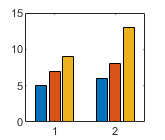'stacked'

Display each group as one multicolored bar. The length of a bar is the sum of the elements in the group.

If y is a vector, then the result is the same as 'grouped'.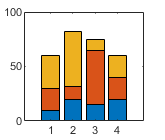'histc'

Display the bars in histogram format, in which the bars in a group touch one another. The trailing edge of each group is aligned with the corresponding x value.

Note

A better way to display a histogram is to call the histogram function.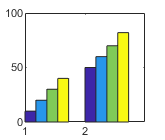'hist'

Display the bars in histogram format. Each group is centered at the corresponding x value.

Note

A better way to display a histogram is to call the histogram function.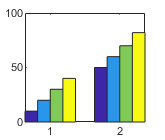Bar color, specified as one of the colors in this table.

ColorColor
'b'Blue
'r'Red
'g'Green
'c'Cyan
'm'Magenta
'y'Yellow
'k'Black
'w'White

Axes object. If you do not specify an axes, then bar uses the current axes for the bar graph.

Name-Value Arguments

Specify optional comma-separated pairs of Name,Value arguments. Name is the argument name and Value is the corresponding value. Name must appear inside quotes. You can specify several name and value pair arguments in any order as Name1,Value1,...,NameN,ValueN.

Example: bar([10 20 30],'EdgeColor','g') specifies a green outline around the bars.

The Bar properties listed here are only a subset. For a complete list, see Bar Properties.

Note

• The properties listed here are only a subset. For a complete list, see Bar Properties.

• You can set these properties only on bar graphs that use the default 'grouped' or 'stacked' style.

Outline color, specified as 'flat', an RGB triplet, a hexadecimal color code, a color name, or a short name. If there are 150 bars or fewer, the default value is [0 0 0], which corresponds to black. If there are more than 150 adjacent bars, the default value is 'none'.

Starting in R2017b, the 'flat' option uses the CData values to color the edges. In previous releases, the 'flat' option colored the edges using colors from the colormap.

For a custom color, specify an RGB triplet or a hexadecimal color code.

• An RGB triplet is a three-element row vector whose elements specify the intensities of the red, green, and blue components of the color. The intensities must be in the range [0,1]; for example, [0.4 0.6 0.7].

• A hexadecimal color code is a character vector or a string scalar that starts with a hash symbol (#) followed by three or six hexadecimal digits, which can range from 0 to F. The values are not case sensitive. Thus, the color codes '#FF8800', '#ff8800', '#F80', and '#f80' are equivalent.

Alternatively, you can specify some common colors by name. This table lists the named color options, the equivalent RGB triplets, and hexadecimal color codes.

Color NameShort NameRGB TripletHexadecimal Color CodeAppearance
'red''r'[1 0 0]'#FF0000''green''g'[0 1 0]'#00FF00''blue''b'[0 0 1]'#0000FF''cyan' 'c'[0 1 1]'#00FFFF''magenta''m'[1 0 1]'#FF00FF''yellow''y'[1 1 0]'#FFFF00''black''k'[0 0 0]'#000000''white''w'[1 1 1]'#FFFFFF''none'Not applicableNot applicableNot applicableNo color

Here are the RGB triplets and hexadecimal color codes for the default colors MATLAB® uses in many types of plots.

[0 0.4470 0.7410]'#0072BD'[0.8500 0.3250 0.0980]'#D95319'[0.9290 0.6940 0.1250]'#EDB120'[0.4940 0.1840 0.5560]'#7E2F8E'[0.4660 0.6740 0.1880]'#77AC30'[0.3010 0.7450 0.9330]'#4DBEEE'[0.6350 0.0780 0.1840]'#A2142F'Example: b = bar(1:10,'EdgeColor','red')

Example: b.EdgeColor = [0 0.5 0.5];

Example: b.EdgeColor = 'flat';

Example: b.EdgeColor = '#D2F9A7';

Fill color, specified as 'flat', an RGB triplet, a hexadecimal color code, a color name, or a short name. The 'flat' option uses the CData property value of the Bar object to color the faces.

For a custom color, specify an RGB triplet or a hexadecimal color code.

• An RGB triplet is a three-element row vector whose elements specify the intensities of the red, green, and blue components of the color. The intensities must be in the range [0,1]; for example, [0.4 0.6 0.7].

• A hexadecimal color code is a character vector or a string scalar that starts with a hash symbol (#) followed by three or six hexadecimal digits, which can range from 0 to F. The values are not case sensitive. Thus, the color codes '#FF8800', '#ff8800', '#F80', and '#f80' are equivalent.

Alternatively, you can specify some common colors by name. This table lists the named color options, the equivalent RGB triplets, and hexadecimal color codes.

Color NameShort NameRGB TripletHexadecimal Color CodeAppearance
'red''r'[1 0 0]'#FF0000''green''g'[0 1 0]'#00FF00''blue''b'[0 0 1]'#0000FF''cyan' 'c'[0 1 1]'#00FFFF''magenta''m'[1 0 1]'#FF00FF''yellow''y'[1 1 0]'#FFFF00''black''k'[0 0 0]'#000000''white''w'[1 1 1]'#FFFFFF''none'Not applicableNot applicableNot applicableNo color

Here are the RGB triplets and hexadecimal color codes for the default colors MATLAB uses in many types of plots.

[0 0.4470 0.7410]'#0072BD'[0.8500 0.3250 0.0980]'#D95319'[0.9290 0.6940 0.1250]'#EDB120'[0.4940 0.1840 0.5560]'#7E2F8E'[0.4660 0.6740 0.1880]'#77AC30'[0.3010 0.7450 0.9330]'#4DBEEE'[0.6350 0.0780 0.1840]'#A2142F'Starting in R2017b, the default value is an RGB triplet from the ColorOrder property of the axes. In previous releases, the default value was 'flat' and the colors were based on the colormap.

Example: b = bar(1:10,'FaceColor','red')

Example: b.FaceColor = [0 0.5 0.5];

Example: b.FaceColor = 'flat';

Example: b.FaceColor = '#D2F9A7';

Color data, specified as one of these values:

• RGB triplet — Single RGB color value applies to all bars.

• Three-column matrix — One color per bar. Each row in the matrix specifies an RGB triplet for a particular bar.

• Scalar — Single color applies to all bars, where the color comes from the colormap.

• Vector — One color per bar. The colors come from the colormap.

By default, when you create a bar chart, the CData property contains a three-column matrix of RGB triplets. You can change the color for a particular bar by changing the corresponding row in the matrix.

This property applies only when the FaceColor or EdgeColor property is set to 'flat'.

Example

Change the color for a particular bar by setting the FaceColor property to 'flat'. Then change the corresponding row in the CData matrix to the new RGB triplet. For example, change the color of the second bar.

b = bar(1:10,'FaceColor','flat');
b.CData(2,:) = [0 0.8 0.8];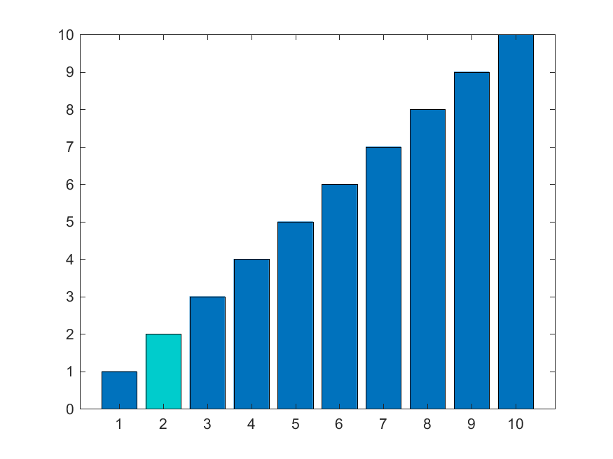Baseline value, specified as a numeric scalar value.

The baseline value that you specify applies to either the x-axis or the y-axis depending on the bar chart orientation. If you change the orientation of the bar chart from vertical to horizontal, or vice versa, the baseline value might change. Set the BaseValue property after setting the Horizontal property.

Line style of bar outlines, specified as one of the line styles in this table.

Line StyleDescriptionResulting Line
'-'Solid line'--'Dashed line':'Dotted line'-.'Dash-dotted line'none'No lineNo line

Width of bar outlines, specified as a positive value in point units. One point equals 1/72 inch.

Example: 1.5

Data Types: single | double | int8 | int16 | int32 | int64 | uint8 | uint16 | uint32 | uint64

Output Arguments

collapse all

Bar objects. Use the elements in b to access and modify properties of a specific Bar object after it has been created. The number of Bar objects depends on the size of y. If y is a vector, then b is one Bar object. If y is a matrix, then b is a vector containing a Bar object for each series in y.

collapse all

Series of Bars

A series consists of the bars at all locations in X for a particular set of data. By default, each series of bars is indicated by a different color.

Group of Bars

A group consists of all the bars at a particular location in X.